College Physics : Resistors

Example Questions

Example Question #1 : Circuits

You are given three resistors with known values: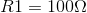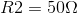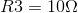You are asked to create a circuit with a total resistance of between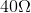and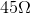. How should you arrange the resistors to accomplish this?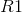and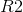in parallel, connected to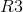in series,, andin series,, andin parallelandin parallel, connected toin seriesandin parallel;is not necessaryandin parallel, connected toin series

Explanation:

This question requires no math to correctly answer! You should not need to 'brute force' it. Although it is designed to appear time consuming, it should be relatively easily once the principle of resistors in parallel is understood. Whenever two resistors are connected in parallel, the net resistance must be less than the resistance of either of the two alone. When resistors are connected in series, the net resistance must be more than the resistance of either alone.

Explanation of correct answer:andin parallel, connected toin series - It is possible to 'eyeball' this to see that this is at least feasible.andin parallel must make a network with an overall resistance less than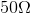. When added in series with(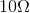), the overall may fall betweenand. To confirm, one could do the math to calculate the overall resistance, but the point of this question is to use general principles to quickly eliminate the other, incorrect answer choices.

Explanations of incorrect answers:,, andin parallel - This combination cannot possibly work since the overall resistance must be less than(the smallest resistor in parallel).andin parallel, connected toin series - Regardless of the overall resistance ofandin parallel, the connection within series makes the total resistance more than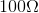.andin parallel;is not necessary - Placingandin parallel must result in a resistance less than.,, andin series - Connecting resistors in series results in an overall resistance greater than that of any one alone. Sinceandare included in series, the sum of the resistances is obviously much greater than what we are asked to produce and this choice can be immediately eliminated.

All College Physics Resources